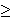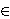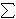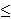# Set Partition Problems

This is my first post of 2019 and my first post in a while. There was one posted a few months ago, but not really geared towards the algorithms and learning focus of the site. I have been doing a lot of coding in my spare time, but honestly life has just gotten in the way. Its not a bad thing, but life is life and sometimes I have to prioritize the things. In particular, I have been having ideas and actually coding things up but the time it takes to clean up code, write a blog entry and finding a nice way to visualize these things has been something that I haven’t been able to really focus on as much as I’ve wanted to.

That said, I want to talk to you about the Set Partition Problem today. You can go to Wikipedia to get more information about this problem, but I will give you a brief introduction to it and then talk about two different approaches to it. The problem assumes that we are given as input a (multi)-set S. The reason we say it is a multi-set and not a simple set is because we can have the same element appear multiple times in the set. So if S1 = {2} and S2 = {2, 2}, then although as sets they are both equal to the set {2} = S1, as multi-sets allow for multiple instances of an element. The elements of S are assumed to be positive integers.

So given this multi-set S, we ask the question of can the elements of S be divided into two smaller multi-sets, C1 and C2 where

• C1 [union] C2 = S
• C1 [intersect] C2 = [empty set]
• [Sigma]_[x in C1] = [Sigma]_[x in C2].C1 [union] C2 = S, C1 [intersect] C2 = [empty set], and [Sigma]_[x in C1] = [Sigma]_[x in C2]

The first two bullets above say that the sets C1 and C2 form a partition of S. The third bullet says that the sums of the elements in the two children multi-sets are equal.

This problem is known to be NP Complete. This means that it is one of the more difficult decision problems. Because of this finding an algorithm that solves this problem exactly will generally take a long running time. And finding an algorithm that runs quickly will more than likely be incorrect in some instances.

I will show you two approaches to this problem. One is based on Dynamic Programming (DP) and one is based on Greedy Algorithms. The DP version solves the problem exactly but has a slow running time. The greedy algorithm is fast but is not guaranteed to always give the correct answer.

Dynamic Programming is based on the principle of optimality. This says that in order to have a correct solution to the overall problem, we need optimal solutions to all subproblems of this problem. This is done by keeping track of a table which can be used for looking up these subproblems and the optimal solutions to these subproblems.

For this problem, we will build a table where the rows represent the final sums and columns represent subsets of the given multi-set (containing the first 0…n elements). The question we are repeatedly asking is “can we find a subset of the set in this column whose sum is exactly the given rowsum?” Here is an example of the DP algorithm on the multi-set {5, 6, 5, 6, 7}.

Greedy Algorithms are generally based on sorting the elements based on some principle and using that to try to answer the underlying question. The main problem with this approach is that it is very short sided because they do not look at the overall picture. Such algorithms are known for finding local optima that are not always globally optimal. The benefit to these problems though is that they are generally easier to code and easier to understand.

For the Set Partition Problem , the Greedy approach is to sort elements in descending order. Once this is done, the goal is to keep two subsets while iterating through the array, adding the element to the smaller of the two sets whenever possible.

For more examples, check out Set Partition Problems

# Approximating the Set Cover ProblemI just finished my weekly task of shopping for groceries. This can be a somewhat daunting task because I generally have a list of things that I’ll need which cannot all be purchased at a single location. What often happens is that I find that many of the items on my list are ONLY offered at certain stores – generic brands of certain items for example. My goal then changes from minimizing the total amount of money spent to minimizing the number of stores that I must visit to purchase all of my items.

To formulate this as a mathematical problem, suppose that I have a grocery list of items I would like to buy, represented by the lists item1, item2, …, itemn, where n represents the number of items I have on this list. Suppose also that there are stores Store1, Store2, …, Storem (each one distinct) that offer some combination of items I have on my list. What I would like to do is minimize the number of stores I have to visit to purchase these items.

The problem I just described is famous because it is one that many people face on a regular basis. In a more general form, it is so famous that it has a name for it, called the Set Cover Problem (or the Minimum Set Cover Problem). In the general form of this problem, we replace the grocery list with a set of items called our universe. The lists of items offered at each store are the collections of subsets of the universe. In the problem, as in the example above, we would like to select enough subsets from this collection that we are able to obtain every element in our universe. We would like to do this with as low a number of sets as possible.

In my previous post, I described the 21 problems that Karp proved were NP-Complete. Set Cover was one of those problems, showing that this is a hard problem to solve. What I will do is introduce three ways to reach a near-optimal solution relatively quickly.

Greedy Method

One of the first approaches one may take to solve this problem is to repeatedly select the subset that contains the most new items. That’s how the greedy approach to set cover operates. The method knows to terminate when all elements belong to one of the selected sets. In the shopping example above, this would be accomplished by visiting the store that had the most items on my list and purchasing those items at this store. Once this is done, the items that have been purchased can be crossed off my list and we can visit the store with the most items on my remaining list, stopping when the list is empty.

Linear Programming Relaxation

Instead of stating the set cover problem with words, there is a way of describing the situation with mathematical inequalities. For instance, suppose that the soap I like to purchase is only available at stores Store1, Store4 and Store9. Then I could introduce a variable xi for each store i and the requirement that I purchase this soap can be restated as :

x1 + x4 + x91

Because we can either purchase some items or not purchase these items, each variable xi is 0 or 1 (called a binary variable). We can introduce similar constraints for each element in our universe (or on our grocery list). These inequalities (called constraints) have the form:

for each element eU,i | eSi xi1

Our goal of minimizing the number of sets chosen (stores visited) can be stated by the objective function:
minimize1in xi

So the mathematical formulation for this problem can be stated as

minimize1in xi
Subject to
for each element eU,i | eSi xi1
for each set i, xi{0, 1}.

Formulations of this type, where variables are restricted to a finite set (in this case the x variables being either 0 or 1) are called integer programs. Unfortunately, there is no easy way to solve these formulations either. However, there is a related problem which can be solved quickly.

Instead of restricting the x variables to the values of 0 or 1, we could allow them to take on any value within this range, i.e. 0xi1 for each set Si. Doing this converts the problem from an integer programming problem into a linear programming problem (called the LP-Relaxation), which can be solved quickly. The issue with this method though is that the solution obtained by an LP-Relaxation is not guaranteed to be an integer. In this case, how do we interpret the values xi?

Randomized Rounding Method

One approach to dealing with a non-integer solution to the LP-Relaxation is to treat the xi values as probabilities. We can say that xi is the probability that we select set i. This works because each value of xi is in the range of 0 to 1, which is necessary for a probability. We need to repeatedly select sets with their associated probabilities until all elements in our universe are covered. Selecting our sets based on this procedure is the randomized rounding approach.

Deterministic Rounding Method

A second approach to dealing with a non-integer solution to the LP-Relaxation is to base our solution on the most occurring element. If we let f be this frequency (i.e.the number of sets that the most occurring element occurs in), then we can define a solution by selecting set i if the LP=Relaxation solution gives the variable xi a value of at least (1/f).

None of these three approaches is guaranteed to give an optimal solution to an instance of this problem. I will not go into it in this post, but these can all be shown to be within some guaranteed range of the optimal solution, thus making them approximation algorithms.

Hope you enjoy.

# Knapsack ProblemsTo help understand this problem, I want you to think about a common situation in many people’s lives. You have a road trip coming up today and you’ve overslept and are at risk of missing your flight. And to top matters off, you were planning to pack this morning but now do not have the time. You quickly get up and begin to get ready. You grab the first bag you see and quickly try to make decisions on which items to take. In your head you’re trying to perform calculations on things you’ll need for the trip versus things that you can purchase when you get there; things that you need to be able to have a good time versus things you can do without. And to top matters off, you don’t have time to look for your ideal luggage to pack these things. So you have the additional constraint that the items you pick must all fit into this first bag you found this morning.

The situation I described above is a common problem. Even if we ignore the part about the flight, and just concentrate on the problem of trying to put the most valuable set of items in our bag, where each item has its own value and its own size limitations, this is a problem that comes up quite often. The problem is known (in the math, computer science and operations research communities) as the knapsack problem. It is known to be difficult to solve (it is said to be NP-Hard and belongs to a set of problems that are thought to be the most difficult problems within its class). Because of this difficulty, this problem has been well studied.

What I provide in my script are two approaches to solving this problem. The first is a greedy approach, which selects a set of items by iteratively choosing the item with the highest remaining value to size ratio. This approach solves very fast, but can be shown to give sub-optimal solutions.

The second approach is a dynamic programming approach. This algorithm will solves the problem by ordering the items 0, 1, …, n and understanding that in order to have the optimal solution on the first i items, the optimal solution must have been first selected on the fist i-1 items. This algorithm will optimally solve the problem, but it requires the computation of many sub-problems which causes it to run slowly.

Update (4/2/2013): I enjoy this problem so much that I decided to implement two additional approaches to the problem: Linear Programming and Backtracking.

The Linear Programming approach to this problem comes from the understanding that the knapsack problem (as well as any other NP-Complete problem) can be formulated as an Integer Program (this is a mathematical formulation where we seek to maximize a linear objective function subject to a set of linear inequality constraints with the condition that the variables take on integer values). In the instance of the knapsack problem we would introduce a variable xi for each item i; the objective function would be to maximize the total value of items selected. This can be expressed as a linear objective function by taking the sum of the products of the values of each item vi and the variable xi; the only constraint would be the constraint saying that all items fit into the knapsack. This can be expressed as a linear inequality constraint by taking the sum of the products of the weights of each item wi and the variable xi. This sum can be at most the total size of the knapsack. In the integer programming formulation, we either select an item or we do not. This is represented in our formulation by allowing the variable xi = 1 if the item is selected, 0 otherwise.

The LP relaxation of an integer program can be found by dropping the requirements that the variables be integer and replacing them with linear equations. So in the case of the knapsack problem, instead of allowing the variables to only take on values of 0 and 1, we would allow the variables to take on any value in the range of 0 and 1, i.e 0 <= xi <= 1 for each item i. We can then solve this LP to optimality to get a feasible solution to the knapsack problem.The second knapsack approach I implemented today is through backtracking. Similar to the Dynamic Programming approach to this problem, the backtracking approach will find an optimal solution to the problem, but these solutions generally take a long time to compute and are considered computationally inefficient. The algorithm I implemented here first orders the item by their index, then considers the following sub-problems for each item i "What is the best solution I can obtain with this initial solution?". To answer this question, the algorithm begins with an initial solution (initially, the empty set) and a set of unchecked items (initially, all items) and recursively calls itself on sub-problems with an additional item as a part of the initial solution and with this item removed from the unchecked items.So check out my knapsack problem page. I think its a good way to be introduced to the problem itself, as well as some of the techniques that are used in the fields of mathematics, computer science, engineering and operations research.

Other Blogs covering this topic:
Journey to the Four Point Oh